# NCERT Solutions for Class 7 Maths Chapter 15 Visualising Solid Shapes

NCERT Solutions for Class 7 Maths Chapter 15 Visualising Solid Shapes: You have already learnt about the 2D geometry like a circle, square, a rectangle or a rhombus which are measured by length and width. We live in a world where every object that we can see or touch has three dimensions length, width, and height. For example,  a cricket ball, an ice cream cone, a book, room, etc are solid figures which has three dimensions. These objects can be described by 3-dimensions length, width, and height. In CBSE NCERT solutions for class 7 maths chapter 15 visualising solid shapes, you will get questions related to identifying the solid shapes and drawing isometric sketches for solid shapes. When you see 3-dimensional objects from different positions or different angles it looks different. In solutions of NCERT for class 7 maths chapter 15 visualising solid shapes, you will get many questions related  to isometric view of solid shape from different sides and angles which will give you more clarity of the concept. Let's understand this chapter with some basic solid shapes.

Cube:- Below given shape is known as a cube. It has equal length, breadth, and height. It consists of 6 faces, 12 edges and 8 vertices. Dice is an example of a cube.Cylinder:- Below given shape is known as a cylinder. The horizontal crosssection of the cylinder is rectangular in shape and the vertical cross-section is circular in shape.The shadow of a sphere will be circular in shape, the shadow of a cone will be triangular in shape, the shadow of the cylinder will be rectangular in shape. In simple words, this shadow is known as a plane view of a solid shape. There are 14 questions in 4 exercises of this chapter. You will get detailed explanations of all these questions in the CBSE NCERT solutions for class 7 maths chapter 15 visualising solid shapes. You can get NCERT solutions from class 6 to 12 for science and maths by clicking on the above link.

The main topics of the NCERT Solutions for Class 7 Maths Chapter 15 Visualising Solid Shapes are:

15.1 Introduction: Plane Figures And Solid Shapes

15.2 Faces, Edges And Vertices

15.3 Nets For Building 3-d Shapes

15.4 Drawing Solids On A Flat Surface

15.4.1 Oblique Sketches

15.4.2 Isometric Sketches

15.5 Viewing Different Sections Of A Solid

15.5.1 One Way To View An Object Is By Cutting Or Slicing

15.5.2 Another Way Is By Shadow Play

15.5.3 A Third Way Is By Looking At It From Certain Angles To Get Different Views

Doing all the questions and activities of Solutions for NCERT Class 7 Maths Chapter 15 Visualising Solid Shapes will be interesting.

## Solutions of NCERT for class 7 maths chapter 15 visualising solid shapes topic 15.1 introduction: plane figures and solid shapes Question:1 Match the shape with the name: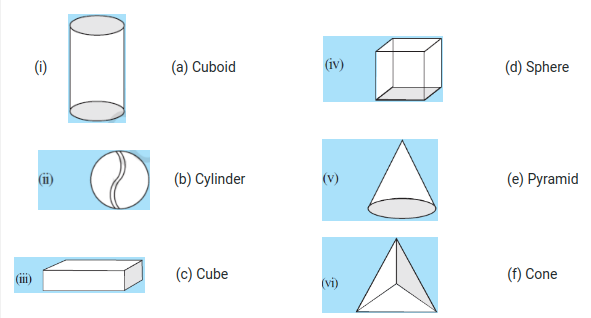These are the correct matches

## CBSE NCERT solutions for class 7 maths chapter 15 visualising solid shapes topic 15.2 faces, edges and vertices

Question: Complete the following table: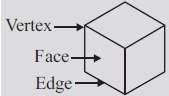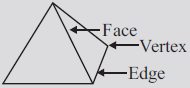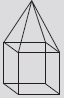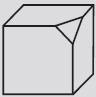Faces (F) 6 4 Edges (E) 12 Vertices (V) 8 4Faces(F)

6

4

10

7

Edges(E)

12

6

16

15

Vertices(V)

8

4

9

10

NCERT solutions for class 7 maths chapter 15 visualising solid shapes topic 15.3 nets for building 3-D shapes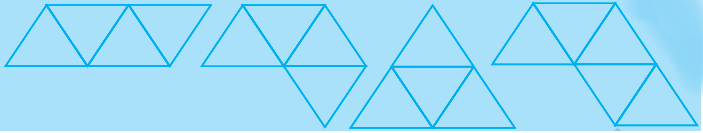Out of these four nets, we can make a  tetrahedron with net (i) and (iii)

Solutions of NCERT for class 7 maths chapter 15 visualising solid shapes-Exercise :15.1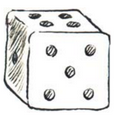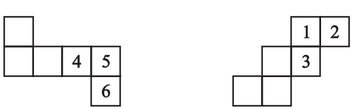Insert suitable numbers in the blanks, remembering that the number on the opposite faces should total to 7.

The sum of the opposite sides of a dice =7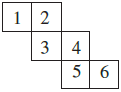NO,
This can not be a net for a die because the opposite faces of a die always have a total sum of 7.
Now, this case one pair is  4  opposite to 1
So, their sum is equal to  5  and not equal to 7
And also, the 2nd pair is 6  opposite to 3 .
So, their sum is equal to 9 and not equal to 7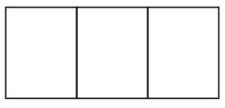There are three faces in the given net. The two possibilities of forming nets areQuestion:5 Match the nets with appropriate solids: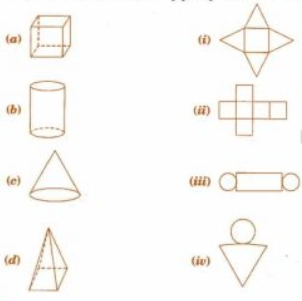Thsese are the correct matches

CBSE NCERT solutions for class 7 maths chapter 15 visualising solid shapes-Exercise :15.2

There are a total of 3 isometric sketches of the cuboid are possible with given dimensions of a cuboidIt is given that three cubes each with 2 cm edge are placed side by side to form a cuboid

So, the oblique sketch of the cuboid is given below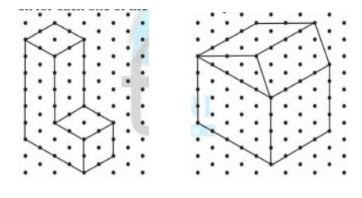Oblique sketch for each one of the given isometric shapes is: -(a) A cuboid of dimensions 5 cm, 3 cm and 2 cm. (Is your sketch unique?)
(b) A cube with an edge 4 cm long.
An isometric sheet is attached at the end of the book. You could try to make on it some cubes or cuboids of  dimensions specified by your friend.The isometric sketch of  s cuboid of dimensions 5 cm, 3 cm and 2 cm. In a similar way, we can draw for the cube alsoNCERT solutions for class 7 maths chapter 15 visualising solid shapes topic 15.4.3 visualising solid objects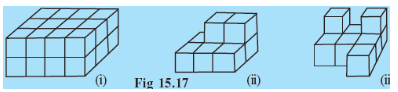i) There are 4 columns and 2 rows in the first line.
So, the total  number of cubes in the first line is

And there is total 3 such line.
Therefore, the total number of cubes are

ii) There are 3 columns and 1 row in the first line at the bottom.
So, the total number of cubes in the first line are

And there are total 2 such lines
So, the total number of cubes at the bottom are
Now, we can see that two cubes are placed on the second line
Therefore, the total number of cubes are

iii)   There are 3 columns and 1 row in the first line at the bottom.
So, the total number of cubes in the first line are

And there are total 2 such lines
So, the total number of cubes at the bottom are
Now, we can see that two cubes are placed on the second line  and one cube in the front of the first line
Therefore, the total number of cubes are

a )  Face opposite to 5 is  2 and opposite to 6  is 1
Therefore, there sum is equal to = 2 + 1 = 3

b )  Face opposite to 4 is 3 and opposite to 3 is 4
Therefore, there sum is equal to = 3 + 4 = 7

Oblique sketch of three cubes each with 2 cm edge  placed side by side to each other to form a cuboid isIn this case
lenght = 2+2+2= 6cm
breath = 2 cm
height = 2cm

Two more cases are possible with

Height = 2+2+2= 6cm
breath = 2 cm
length = 2cm

And

breath = 2+2+2= 6cm
length = 2 cm
height = 2cm

Solutions of NCERT for class 7 maths chapter 15 visualising solid shapes-Exercise :15.3

(i) vertical cut          (ii) horizontal cut

to the following solids?

(a) A brick        (b) A round apple          (c) A die     (d) A circular pipe        (e) An ice cream cone

a )  A brick

i ) Vertical cutA vertical cut gives a square or rectangular crossection

ii )  The horizontal cut  gives rectangular crossectionb ) A round apple

In both, the case crossection will be circular in shape approximatelyc ) A die

both the cut will give a square shaped ) A circular pipe

i ) A vertical cut will give a circular shapeii ) The horizontal cut  gives a rectangular shapee ) An ice cream cone

i) The vertical cut gives a triangular shapeii) The horizontal cut gives a circular shapeNCERT solutions for class 7 maths chapter 15 visualising solid shapes-Exercise :15.4

(i) The cube can cast a shadow in the shape of a rectangle.
(ii) The cube can cast a shadow in the shape of a hexagon.

A cube can cast shadow only in the shape of a square.
Therefore,  both statements (i) and (ii) are not possible

NCERT solutions for class 7 maths chapter 15 visualising solid shapes topic 15.5.3 a third way is by looking at it from certain angles to get different views

For a )

For b)

For c)

For d)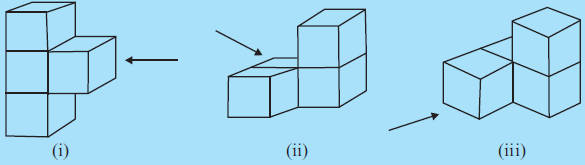Drawn view of each solid as seen from the direction indicated by the arrow are  : -

i )ii )iii )NCERT Solutions for Class 7 Maths- Chapter-wise

 Chapter No. Chapter Name Chapter 1 Solutions of NCERT for class 7 maths chapter 1 Integers Chapter 2 CBSE NCERT solutions for class 7 maths chapter 2 Fractions and Decimals Chapter 3 NCERT solutions for class 7 maths chapter 3 Data Handling Chapter 4 Solutions of NCERT for class 7 maths chapter 4 Simple Equations Chapter 5 CBSE NCERT solutions for class 7 maths chapter 5 Lines and Angles Chapter 6 NCERT solutions for class 7 maths chapter 6 The Triangle and its Properties Chapter 7 Solutions of NCERT for class 7 maths chapter 7 Congruence of Triangles Chapter 8 NCERT solutions for class 7 maths chapter 8 comparing quantities Chapter 9 CBSE NCERT solutions for class 7 maths chapter 9 Rational Numbers Chapter 10 NCERT solutions for class 7 maths chapter 10 Practical Geometry Chapter 11 Solutions of NCERT for class 7 maths chapter 11 Perimeter and Area Chapter 12 CBSE NCERT solutions for class 7 maths chapter 12 Algebraic Expressions Chapter 13 NCERT solutions for class 7 maths chapter 13 Exponents and Powers Chapter 14 Solutions of NCERT for class 7 maths chapter 14 Symmetry Chapter 15 NCERT Solutions for Class 7 Maths Chapter 15 Visualising Solid Shapes

## Benefits of NCERT solutions for class 7 maths chapter 15 visualising solid shapes-

• There are some questions to make an isometric shape using paper which you should try at your home to visualize and understand it better.
• These NCERT solutions will make your homework easy as you will find the detailed explanations of all the NCERT questions including practice questions given below every topic.
• As the name suggests this chapter is more about visualizing and drawing the front view, side view, and top view and the isometric view of the solid shapes. In CBSE NCERT solutions for class 7 maths chapter 15 visualising solid shapes, you will get step-by-step explanations to draw all the projection view from the solid shape and the isometric view from the projected view.

Happy learning!!!